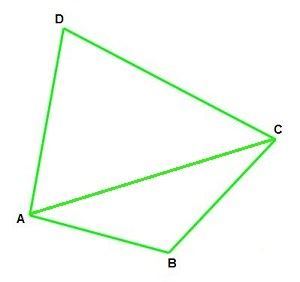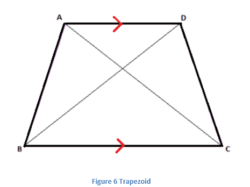## Angle Sum Property of a Quadrilateral

According to the angle sum property of a Quadrilateral, the sum of all the four interior angles is 360 degrees.• ∠ABC, ∠BCD, ∠CDA, and ∠DAB are the internal angles.
• AC is a diagonal

We have learned that the sum of internal angles of a quadrilateral is 360°, that is, ∠ABC + ∠BCD + ∠CDA + ∠DAB = 360°.

let’s prove that the sum of all the four angles of a quadrilateral is 360 degrees.

• We know that the sum of angles in a triangle is 180°.

∠D + ∠DAC + ∠DCA = 180°                           (Sum of angles in a triangle)

• Now consider triangle ABC,

∠B + ∠BAC + ∠BCA = 180°                            (Sum of angles in a triangle)

• On adding both the equations obtained above we have,

(∠D + ∠DAC + ∠DCA) + (∠B + ∠BAC + ∠BCA) = 180° + 180°

∠D + (∠DAC + ∠BAC) + (∠BCA + ∠DCA) + ∠B = 360°

• We see that (∠DAC + ∠BAC) = ∠DAB and (∠BCA + ∠DCA) = ∠BCD.
• Replacing them we have,

∠D + ∠DAB + ∠BCD + ∠B = 360°

• That is,

∠D + ∠A + ∠C + ∠B = 360°.

Or, the sum of angles of a quadrilateral is 360°. This is the angle sum property of quadrilaterals.

A quadrilateral has 4 angles. The sum of its interior angles is 360 degrees. We can find the angles of a quadrilateral if we know 3 angles or 2 angles or 1 angle and 4 lengths of the quadrilateral. In the image given below, a Trapezoid (also a type of Quadrilateral) is shown.

The sum of all the angles ∠A +∠B + ∠C + ∠D = 360°In the case of square and rectangle, the value of all the angles is 90 degrees. Hence,

∠A = ∠B = ∠C = ∠D = 90°

A quadrilateral, in general, has sides of different lengths and angles of different measures. However, squares, rectangles, etc. are special types of quadrilaterals with some of their sides and angles being equal.

Do the Opposite side in a Quadrilateral equals 180 Degrees?

There is no relationship between the opposite side and the angle measures of a quadrilateral. To prove this, the scalene trapezium has the side length of different measure, which does not have opposite angles of 180 degrees. But in case of some cyclic quadrilateral, such as square, isosceles trapezium, rectangle, the opposite angles are supplementary angles. It means that the angles add up to 180 degrees. One pair of opposite quadrilateral angles are equal in the kite and two pair of the opposite angles are equal in the quadrilateral such as rhombus and parallelogram. It means that the sum of the quadrilateral angles is equal to 360 degrees, but it is not necessary that the opposite angles in the quadrilateral should be of 180 degrees.

There are basically five types of quadrilaterals. They are;

1. Parallelogram: Which has opposite sides as equal and parallel to each other.
2. Rectangle: Which has equal opposite sides but all the angles are at 90 degrees.
3. Square: Which all its four sides as equal and angles at 90 degrees.
4. Rhombus: Its a parallelogram with all its sides as equal and its diagonals bisects each other at 90 degrees.
5. Trapezium: Which has only one pair of sides as parallel and the sides may not be equal to each other.

### Example

1. Find the fourth angle of a quadrilateral whose angles are 90°, 45° and 60°.

Solution: By the angle sum property we know;

Sum of all the interior angles of a quadrilateral = 360°

Let the unknown angle be x

So,

90° + 45° + 60° + x = 360°

195° +  x = 360°

x = 360° – 195°

x = 165°